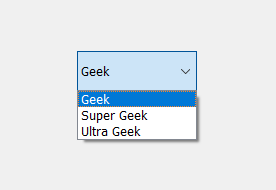Open In App

# PyQt5 – QComboBox

QComboBox is a widget in PyQt5 which is used to choose from a list. It takes minimum space when we click on combo box, a drop down list came from which we can select the item. `QComboBox` class is used to add a combo box in a application. Below is the representation of how combo looks like –Example :
In this we will create a combo box in the window which allows us to choose in between “Geek”, “Super Geek” and “Ultra Geek”

Below is the code –

 `# importing libraries``from` `PyQt5.QtWidgets ``import` `*` `from` `PyQt5 ``import` `QtCore, QtGui``from` `PyQt5.QtGui ``import` `*` `from` `PyQt5.QtCore ``import` `*` `import` `sys`` ` ` ` `class` `Window(QMainWindow):`` ` `    ``def` `__init__(``self``):``        ``super``().__init__()`` ` `        ``# setting title``        ``self``.setWindowTitle(``"Python "``)`` ` `        ``# setting geometry``        ``self``.setGeometry(``100``, ``100``, ``600``, ``400``)`` ` `        ``# calling method``        ``self``.UiComponents()`` ` `        ``# showing all the widgets``        ``self``.show()`` ` `    ``# method for widgets``    ``def` `UiComponents(``self``):`` ` `        ``# creating a combo box widget``        ``combo_box ``=` `QComboBox(``self``)`` ` `        ``# setting geometry of combo box``        ``combo_box.setGeometry(``200``, ``150``, ``120``, ``40``)`` ` `        ``# adding items to combo box``        ``combo_box.addItem(``"Geek"``)``        ``combo_box.addItem(``"Super Geek"``)``        ``combo_box.addItem(``"Ultra Geek"``)`` ` `# create pyqt5 app``App ``=` `QApplication(sys.argv)`` ` `# create the instance of our Window``window ``=` `Window()`` ` `# start the app``sys.exit(App.``exec``())`

Output :